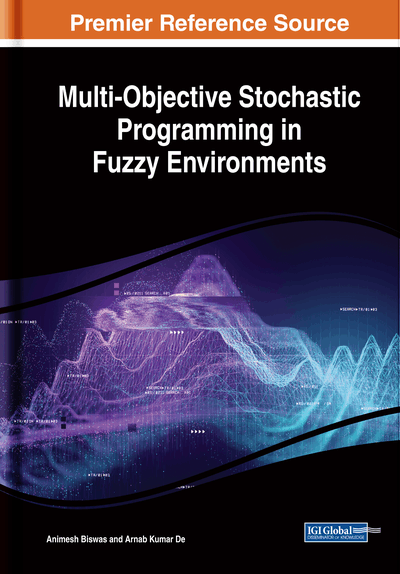# Fuzzy Linear Multi-Objective Stochastic Programming Models

DOI: 10.4018/978-1-5225-8301-1.ch003
Available
\$33.75
List Price: \$37.50
10% Discount:-\$3.75
TOTAL SAVINGS: \$3.75

## Abstract

In this chapter, fuzzy goal programming (FGP) technique is presented to solve fuzzy multi-objective chance constrained programming (CCP) problems having parameters associated with the system constrains following different continuous probability distributions. Also, the parameters of the models are presented in the form of crisp numbers or fuzzy numbers (FNs) or fuzzy random variables (FRVs). In model formulation process, the imprecise probabilistic problem is converted into an equivalent fuzzy programming model by applying CCP methodology and the concept of cuts of FNs, successively. If the parameters of the objectives are in the form of FRVs then expectation model of the objectives are employed to remove the probabilistic nature from multiple objectives. Afterwards, considering the fuzzy nature of the parameters involved with the problem, the model is converted into an equivalent crisp model using two different approaches. The problem can either be decomposed on the basis of tolerance values of the parameters; alternatively, an equivalent deterministic model can be obtained by applying different defuzzification techniques of FNs. In the solution process, the individual optimal value of each objective is found in isolation to construct the fuzzy goals of the objectives. Then the fuzzy goals are transformed into membership goals on the basis of optimum values of each objective. Then priority-based FGP under different priority structures or weighted FGP is used for achievement of the highest membership degree to the extent possible to achieve the ideal point dependent solution in the decision-making context. Finally, several numerical examples considering different types of probability distributions and different forms of FNs are considered to illustrate the developed methodologies elaborately.
Chapter Preview
Top

## 3.1 Fuzzy Chance Constrained Programming Model

In previous chapters, it is already mentioned that CCP methodology is used to solve stochastic programming problems in which the associated parameters are in the form of random variables following some known probability distribution. In 1959, Charnes and Cooper (1959) first introduced the concept of CCP as a tool to solve optimization problems with probabilistic uncertainties. In CCP (Kataoka, 1963; Prekopa, 1973; Sinha et al., 1998) if the problem contains several numbers of conflicting objectives, it is known as multi-objective CCP. Methodological aspects of CCP problems were further studied (Mohammed, 2000) and applied to solve different real life decision making problems by several researchers in the field of watershed nutrient load reduction (Dong et al., 2014), vehicle routine problem (ZareMehrjerdi, 1986), urban land-use planning and land use policy analysis (Zhou, 2015), etc.

Again, in modeling real life problems, a decision may have to be taken on the basis of some data which are not only probabilistic but also possibilistic or a combination of both. This situation occurs due to the lack of decision makers’ sufficient knowledge about the occurrence as well as value of the parameters involved with the process concerned. So the concept of FRVs (Kwakernaak, 1978) was introduced in hybrid decision making environments. Different researchers (Sakawa et al., 2003; Luhandjula and Robert 2010) developed various techniques using FRVs (Lai and Hwang, 1994; Luhandjula, 1996) to solve CCP problems in fuzzy stochastic environment. In 2001, Liu (2001) proposed a simple expected value model with FRVs. An FGP technique for solving CCP with FRVs associated with the system constraints was presented by Biswas and Modak (2011, 2012). A new method for solving multi-objective linear programming models with FRVs is developed by Nematian (2012). Yano and Sakawa (2012) proposed an interactive method for solving multi-objective fuzzy random linear programming problem through fractile criteria. In 2017 Katagiri et al. (2017) developed a necessity measure based probabilistic expectation models for linear programming problems with discrete FRVs. Recently, De et al. (2018), formulated a methodology for solving multi-objective fuzzy stochastic programming problems with Cauchy and extreme value distributed FRVs.

It has been found that most of the parameters of probabilistic models assume normal distribution (Hock and Schittkowski, 1981) and some of them follow exponential distribution (Biswal et al, 1998) also. During the last decade stochastic linear programming with Cauchy distribution and extreme value distribution was investigated by Sahoo and Biswal (2005). In their method two multi-objective linear programming models are considered with right side parameters of the constraints of one model as Cauchy distributed random variables and another as extreme value distributed random variables. Finally, to find the compromise solution goal programming methodology is used for both the models. Like various probability distributions Cauchy distribution provides distribution of ratio of two independent standardized normal variates whereas extreme value distribution presents a limiting model for the distribution of the maximum or minimum of finite number of values selected from an exponential type distribution such as normal, gamma, exponential. Also log-normal distribution plays an important role for its wide applications in the fields of engineering, earth sciences, medicines, radioactivity and other decision making arena. The genesis of log normal distribution is found during nineteenth century in the work of Galton (1879), Weber (1834), Fechner (1897) and others.

## Complete Chapter List

Search this Book:
Reset